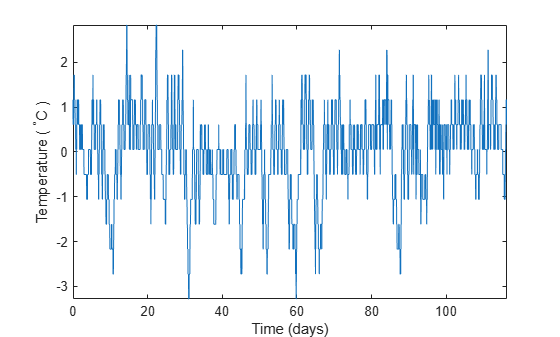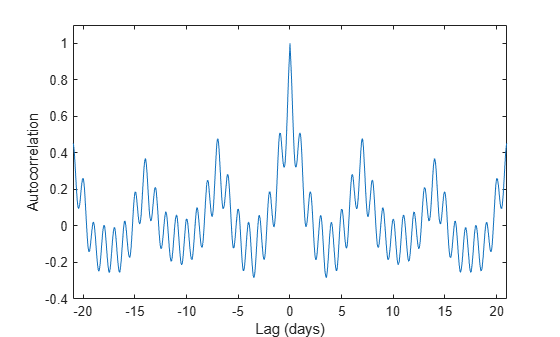# 使用自相关求周期性

```load officetemp tempC = (temp-32)*5/9; tempnorm = tempC-mean(tempC); fs = 2*24; t = (0:length(tempnorm) - 1)/fs; plot(t,tempnorm) xlabel('Time (days)') ylabel('Temperature ( {}^\circC )') axis tight``````[autocor,lags] = xcorr(tempnorm,3*7*fs,'coeff'); plot(lags/fs,autocor) xlabel('Lag (days)') ylabel('Autocorrelation') axis([-21 21 -0.4 1.1])``````[pksh,lcsh] = findpeaks(autocor); short = mean(diff(lcsh))/fs```
```short = 1.0021 ```
```[pklg,lclg] = findpeaks(autocor, ... 'MinPeakDistance',ceil(short)*fs,'MinPeakheight',0.3); long = mean(diff(lclg))/fs```
```long = 6.9896 ```
```hold on pks = plot(lags(lcsh)/fs,pksh,'or', ... lags(lclg)/fs,pklg+0.05,'vk'); hold off legend(pks,[repmat('Period: ',[2 1]) num2str([short;long],0)]) axis([-21 21 -0.4 1.1])```